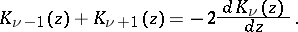# Macdonald function

modified cylinder function, Bessel function of imaginary argument

A function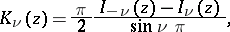whereis an arbitrary non-integral real number and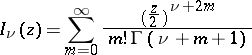is a cylinder function with pure imaginary argument (cf. Cylinder functions). They have been discussed by H.M. Macdonald . Ifis an integer, thenThe Macdonald functionis the solution of the differential equation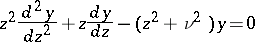(*)

that tends exponentially to zero as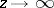and takes positive values. The functionsandform a fundamental system of solutions of (*).

For,has roots only when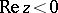. If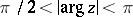, then the number of roots in these two sectors is equal to the even number nearest to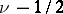, provided thatis not an integer; in the latter case the number of roots is equal to. For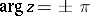there are no roots ifis not an integer.

Series and asymptotic representations are:whereis a non-negative integer;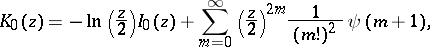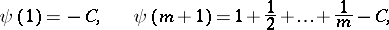where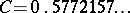is the Euler constant;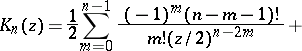where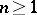is an integer;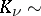for largeand.

Recurrence formulas: